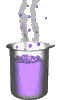Science class online
Chemistry and physics lessons

# Mechanics

Free online mechanics lesson for elementary school, middle school and high school._____________________________________________________   _____________________________________________________

## Gravitationnal acceleration

### g value on the surface of some planets and stars

 celestial object g (N/kg) Sun 273,95 Mercury 3,70 Venus 8.87 Earth 9.8108 Moon 1.62 Mars 3.71 Jupiter 24,79 Saturn 10,44 Uranus 8,87 Neptune 11,15 Pluto 0,66

______________________________________

______________________________________

### Unit and notation

It is noted g, always in tiny letter, not to be confused with "G", in capital letter, which represents the universal gravitational constant.

Its unit is Newton per kilogram, symbol N / kg or N.kg-1

This unit can easily be found using the relation g = P: m where it clearly appears that the Gravitational Field Intensity corresponds to the ratio of a force (in Newton) by a mass (in kg).

Note: The Gravitational Field Intensity is equivalent to an acceleration (sometimes called gravitational acceleration), so it is also possible to express it in meter per second squared (m/s2 or m.s-2).

### Definition

The Gravitational acceleration is defined in the vicinity of a celestial object as the coefficient of proportionality between the mass of a system and the force intensity (the weight) applied by the celestial object on this system. Thus for a system of mass m, of weight P on a given celestial object where the Gravitational Field Intensity is noted g (celestial object) we can use the following relation:

P = m. g(celestial object)

### Gravitational acceleration Intensity and gravitation

The weight results from the gravitation force but also from the inertia force resulting from the rotation of the celestial object, but they are most often negligible. therefore, we consider that the Gravitational Field Intensity is related to gravitation, and most often we calculate the value of the Gravitational Field Intensity using gravitational force.

______________________________________

______________________________________

### g expression on any celestial object

On the surface of a celestial object with a mass mA and a radius RA, the gravitational force applied on an object with a mass m:
 F = G.mA.m RA2

If the gravitational force is assimilated to the weight with the expression:
P = gA. m

Then the expression of the Gravitational Field Intensity on the surface of the celestial object A is:
 gA = G.mA RA2
Example
On planet Earth with the mass is MT = 5.97 x 1024 kg and its average radius RT = 6370 km:

 gT = 6,67.1011.5.97 x 1024 (6370.103)2

gT = 9,81 N/kg

### Variations of g

The Gravitational Field Intensity depends on the same factors as the gravitational force:
• The mass of the celestial object.
• The distance from the center of the celestial object

This implies that the Gravitational Field Intensity:
• depends on the celestial object (and its mass)
• decreases with increasing altitude

______________________________________

______________________________________

### Interval in which g can be considered as a constant

g depends on the altitude but can be considered as a constant over a certain height. We take the example of the Earth and its average radius of 6370 km. If we consider a point located at an altitude of 1 km, that is 6371 km from the center then the expression of g does not vary and remains 9.81 N / kg.

If we consider a point located at an altitude of 10 km (at 6380 km of the center) then we obtain 9.78 N / kg, and for a point situated at 100 km we obtain g = 9.51 N / kg. Therefore, on Earth:

• The value of g expressed to the nearest hundredth (9.81 N / kg) can be considered as a constant for altitudes with values ​​of the order of km.
• g, expressed to the nearest tenth (9.8 N / kg), can be considered as a constant for altitudes with values ​​of the order of ten km.
• g, expressed to the nearest unit (10 N / kg), can be considered as a constant for altitudes with values ​​of the order of hundred km

### Gravity field

We can associate to the Gravitational Field Intensity to a scalar quantity making possible the definition of the Gravitational Field Intensity. At a given point, the vector has the same value as g, same direction and same meaning as the weight vector: vertical and downward, and to be more precise towards the center of the celestial object. Over a limited area (in extent and in altitude) the gravity field may be considered uniform, i.e. the vector g is constant and the field lines are parallel.

______________________________________

______________________________________

____________________________________

____________________________________